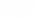## 暴击几率

### 相对增加

= 0.3576 = 35.76%

= 0.75 = 75.00%

## 暴击倍率

• 武器基础暴击倍率: 大多数的武器的固有暴击倍率2.0x。
• MOD的暴击倍率增益: 该增益为当前所装备的所有MOD带来的暴击倍率增益总和。当同时装备满级的和后(具体数值为1.20和0.60)，所产生的MOD暴击倍率增益为1.80。

## 暴击等级

x = 0% 没有攻击能够暴击 Tier 0
0% < x < 100% 有几率触发 暴击
Tier 1
x = 100% 所有攻击均能触发黄色暴击
100% < x < 200% 有几率触发强力暴击
Tier 2
x = 200% 所有攻击均能触发橙色暴击
200% < x < 300% 有几率触发 超级暴击
Tier 3
x = 300% 所有攻击均能触发红色暴击
300% < x < 400% 有几率触发红色暴击(tier 4)
Tier 4
x = 400% 所有攻击均能触发红色暴击(tier 4)
400% < x < 500% 有几率触发 红色暴击 (tier 5)
...
... ...

• 简单来说：每提升一个暴击等级，会有额外的暴击倍率提升，从而提高暴击伤害。
• 所以一般而言 红色暴击伤害>橙色暴击伤害>黄色暴击伤害。但是实际情况可能有所不同。
• 所有可能性情况均基于上一层概率计算暴击几率 概率达到的暴击等级 保底达到的暴击等级 0.01% − 100% 1 N/A 100.01% − 200% 2 1 200.01% − 300% 3 2 300.01% − 400% 4 3 400.01% − 500% 5 4 500.01% − 600% 6 5 600.01% − 700% 7 6 700.01% − 800% 8 7 ••• ••• ••• n% < 暴击几率 ≤ (n% + 100%) (n% + 100%) ÷ 100 (n% + 100%) ÷ 100-1

• 超过300%暴击几率以后出现全是红色暴击。

## 爆头暴击

• 爆头弱点倍率: 绝大多数情况下为敌人头部受到攻击时所得到的伤害加成（恐鸟及其变种的头部位置比较特殊），倍率一般为 2 倍。可受锁定目标+60%爆头倍率、守望者开镜增加爆头伤害等影响。但特殊武器如捕月、雷霆、弧电离子枪因特殊算法导致此项无效，此时应计算为1倍。
• 面板暴击伤害: 与前面提到的“暴击倍率”相同，它是武器固有暴击倍率和MOD带来的暴击倍率加成的总和。在军械库中将选择对应武器后即可在面板上看到这个信息。

## 暴击伤害潜力

### 对伤害潜力的影响

• 不爆头平均伤害 = [ 暴击几率 × （暴击伤害 - 1） + 1 ] × 伤害为什么？这里是证明
• 爆头平均伤害 = 爆头倍率 × [ 暴击几率 × （暴击伤害 × 2 - 1） + 1 ] × 伤害

• 平均伤害 = 爆头几率 × 爆头平均伤害 + （1 - 爆头几率）× 不爆头平均伤害
• 爆头几率 根据玩家的实际射击情况进行估算，即通常一定射击次数下发生爆头的次数与射击次数的比值。

$\frac{d}{dp}(m (3 n - 1) + 1 - \frac{2mn}{1+p}) = \frac{2mn}{(1+p)^2}$

### 暴击相关MOD的增益

• czm: 暴击区倍率。
• p: 爆头几率，1.00表示每发均爆头，0表示没有一发爆头。
• m: 当前暴击几率，基础暴击几率 × (1 + 暴击几率加成)。
• n: 当前暴击倍率，基础暴击倍率 × (1 + 暴击倍率加成)。
• v: 当前暴头倍率，2 × (1 + 暴头倍率加成)。

### 公式证明

$m = mod(x)$ 几率造成第 $n = ceil(x)$ 级暴击

$=y(m + n - 1) - (m + n - 1) + 1$

$=xy - x + 1$

$=x(y - 1) + 1$

## 更新历史

• 增加了橙色暴击指示！现在，当暴击发生于100%至200%区间时，显示的是橙色暴击。而当暴击发生在200%以上时，显示的才是红色暴击！这仅仅是针对暴击伤害显示的改动，不会对伤害本身有任何影响。
5.0
2人评价
0

2年
0

(n% + 100%) ÷ 100=（n+100)/10000=暴击等级？我理解错了？

2年
0

2年
0

2年
0

cr: 面板暴击倍率
cp: 面板暴击概率
crit_lvl: 暴击等级
ratio1: 运气不好的伤害倍率(未达到下一暴击等级)
ratio2: 运气好的伤害倍率(达到了下一暴击等级)

crit_lvl = floor(cp)
cp' = cp - crit_lvl
ratio1 = 1 + (cr - 1) *  crit_lvl
ratio2 = 1 + (cr - 1) * (crit_lvl + 1)
total_damage_ratio = ratio1 * (1 - cp') + ratio2 * cp'

......

......

total_damage_ratio = (cr - 1) * cp + 1

2年
0

2年
0

2年
Lysinelai
0

2年
0

黄色暴击伤害/爆头倍率/暴击爆头倍率/暴击倍率=1810/2/2/2=226.25
橙色暴击伤害/爆头倍率/暴击爆头倍率/橙色暴击倍率=3168/2/2/[2*（2-1）+1]=3168/2/2/3=264(从橙字开始公式就不对了)

2年
0

2年
Lysinelai
0

2年
0

2年
Div2009
1

2年
0

2年
Smile slime 47
0

2年
0

2年
0

2年
Div2009
0

3年